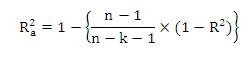# Multiple Regression Correlation Coefficient - Definition & Meaning

Published in Statistics by MBA Skool Team

## What is Multiple Regression Correlation Coefficient ?

R2, or coefficient of determination, as it is also called, is a tester parameter of simple and multiple regression models. In multiple regression models, R2 represents how much the independent variables can explain the behaviour of the dependent variable. Thus, R2 represents the explanatory power of a regression model.

In simple regression with one independent variable, R2 is simply the square of the correlation coefficient.

In multiple linear regression with more than one explanatory variables, with the intercept of the regression straight line included, R2 is called the multiple correlation coefficient.

In all the standard statistical solvers including MS Excel, R2 is provided in the output. For example, an R2 of 74% from the solver output implies the regression model can explain 74% of the behaviour of the dependent variable. This indicates we may refine our regression model a bit.

However, a problem with R2 is that it increases as one simply plugs in more independent variables into the regression model, even if they do not increase the explanatory power of the model. To solve this problem, adjusted R2 (Ra2) is used (also provided by all statistical solvers) defined below, which is free from this bias.where, n = no. of observations, k = no. of independent variables.

Hence, this concludes the definition of Multiple Regression Correlation Coefficient along with its overview.

This article has been researched & authored by the Business Concepts Team. It has been reviewed & published by the MBA Skool Team. The content on MBA Skool has been created for educational & academic purpose only.

Browse the definition and meaning of more similar terms. The Management Dictionary covers over 1800 business concepts from 5 categories.